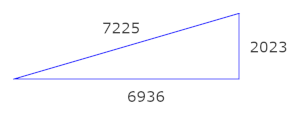# Pythagorean triangles with side 2023

Can a Pythagorean triangle have one size of length 2023? Yes, one possibility is a triangle with sides (2023, 6936, 7225).Where did that come from? And can we be more systematic, listing all Pythagorean triangles with a side of length 2023?

Euclid’s formula generates Pythagorean triples by sticking integers m and n into the formula

(m² – n², 2mn, m² + n²).

If one of these three numbers equals 2023, it’s going to have to be the first one. Why? The second number is even, and 2023 is odd, so that one is ruled out.

It’s less obvious but true that the hypotenuse m² + n² cannot be 2023. We could verify this by brute force, trying integer values of m between 1 and √2023, subtracting m² from 2023, and seeing whether the result is a square. But there is a more elegant approach.

There is a theorem that says an integer N is the sum of two squares exactly when every prime that divides N is either congruent to 1 mod 4 or appears an even number of times in the factorization of N. The prime factorization of N is 7 × 17 × 17. The factor 7 appears to an odd power and 7 = 3 mod 4.

Is 2023 a difference of squares? Yes, every odd number is a difference of squares.

To write an number N as a difference of squares, we have to solve

m² – n² = (m + n)(m – n) = N.

If N is odd, we can always set mn = 1. In the case N = 2023, this means m = 1012 and n = 1011.

The solution above corresponds to factoring 2023 as 2023 × 1. We could also factor 2023 as 289 × 7 or 119 × 17. (The first factor has to be larger than the second for mn to be positive.) To find more possible value of m and n we solve the equations

m + n = 289
mn = 7

and

m + n = 119
mn = 17.

These have solution (148, 141) and (68, 51).

To summarize, we can find three Pythagorean triples with one component equal to 2023 using Euclid’s formula. These are

(2023, 2046264, 2046265)
(2023, 41736, 41785)
(2023, 6936, 7225)

corresponding to (m, n) = (1013, 1012), (148, 141), and (68, 51) respectively. The first two are primitive Pythagorean triples because the three numbers are relative prime in both cases. The last triple is not primitive because each number is divisible by 289.

Euclid’s formula generates all primitive Pythagorean triples, but it doesn’t generate all possible Pythagorean triples. To find all Pythagorean triples we have to consider solutions of the form

(km² – kn², 2kmn, km² + kn²)

for some integer k. If 2023 is k times a difference of squares, k must be a factor of 2023, and so k = 1, 7, 17, 119, 289, or 2023. We’ve already considered the case k = 1.

For k = 7, we express 289 as a difference of squares. The only solutions with positive integers is (m, n) = (145, 144). This yields the Pythagorean triple

(2023, 292320, 292327).

For k = 17, we express 119 as a difference of squares. The solutions are (m, n) = (60, 59) and (12, 5). These yield

(2023, 120360, 120377)

and

(2023, 2040, 2873).

For k = 119, we express 17 as a difference of squares. This can only be done one way, (m, n) = (9, 8). This yields

(2023, 17136, 17255).

For k = 289, we express 7 as a difference of squares. The unique solution (4, 3) yields the triple

(2023, 6936, 7225)

For k = 2023, we have to write 1 as a difference of squares. This has no solution if we require both squares to be positive.

So we’re done. We’ve found all Pythagorean triples containing 2023.

## One thought on “Pythagorean triangles with side 2023”

1. 2023 cannot be the hypotenuse of a primitive Pythagorean triple
but 17 = 16 + 1 so
7*17*8, 7*17*15, 7*17*17 =2023 is and
289 = 225 + 64 so
7*(225-64),7*2*15*8, 7 *289 = 2023 works